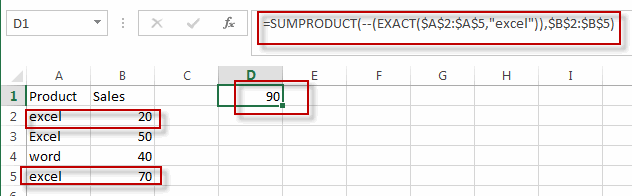# Sum Cell Value with Case Sensitive

This post will guide you how to sum cell values based on a specific value with case sensitive in excel. How do I sum cell values with case sensitive with a formula in excel. How to sum cell values based on a criteria that is sensitive in excel.

## Sum Cell Value with Case Sensitive

Assuming that you have a list of data in the range A1:B5, and you want to sum cell values based on “excel” product only, and ignore “Excel”. So you can create a new formula based on the SUMPRODUCT function and the EXACT function.

You just need to type the following formula into the cell D1, then press enter key to apply this formula.

`=SUMPRODUCT(--(EXACT(\$A\$2:\$A\$5,"excel")),\$B\$2:\$B\$5)`

Let’s see the result:### Related Functions

• Excel SUMPRODUCT function
The Excel SUMPRODUCT function multiplies corresponding components in the given one or more arrays or ranges, and returns the sum of those products.The syntax of the SUMPRODUCT function is as below:= SUMPRODUCT (array1,[array2],…)…
• Excel EXACT function
The Excel EXACT function compares if two text strings are the same and returns TRUE if they are the same, Or, it will return FALSE.The syntax of the EXACT function is as below:= EXACT (text1,text2)…